### Home > CALC > Chapter Ch10 > Lesson 10.1.7 > Problem10-69

10-69.
1. Examine the integrals below. Consider the multiple tools available for integrating and use the best strategy. After evaluating each integral, write a short description of your method. Homework Help ✎

1.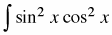2.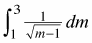3.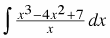4.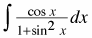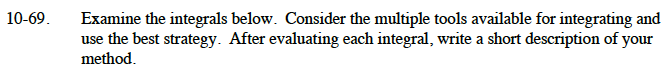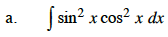$\sin^2(x)\cos^2(x)=\frac{1}{4}\sin^2(2x)$

$=-\frac{1}{8}(2\sin^2(2x))$

$=-\frac{1}{8}(-1+(1-2\sin^2(2x)))$

$=-\frac{1}{8}(-1+\cos(4x))$

Rewrite the integrand using this final expression. You should now be able to complete the integration.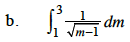$=\left.\frac{\sqrt{m-1}}{1/2}\right|_1^3$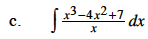$=\int\Big({x^2-4x+\frac{7}{x}}\Big)dx$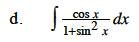Use substitution. Let u = sin(x).

Once the substitution is made, you should recognize the integrand as the derivative of an inverse trigonometric function.